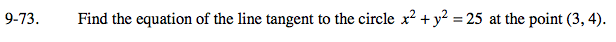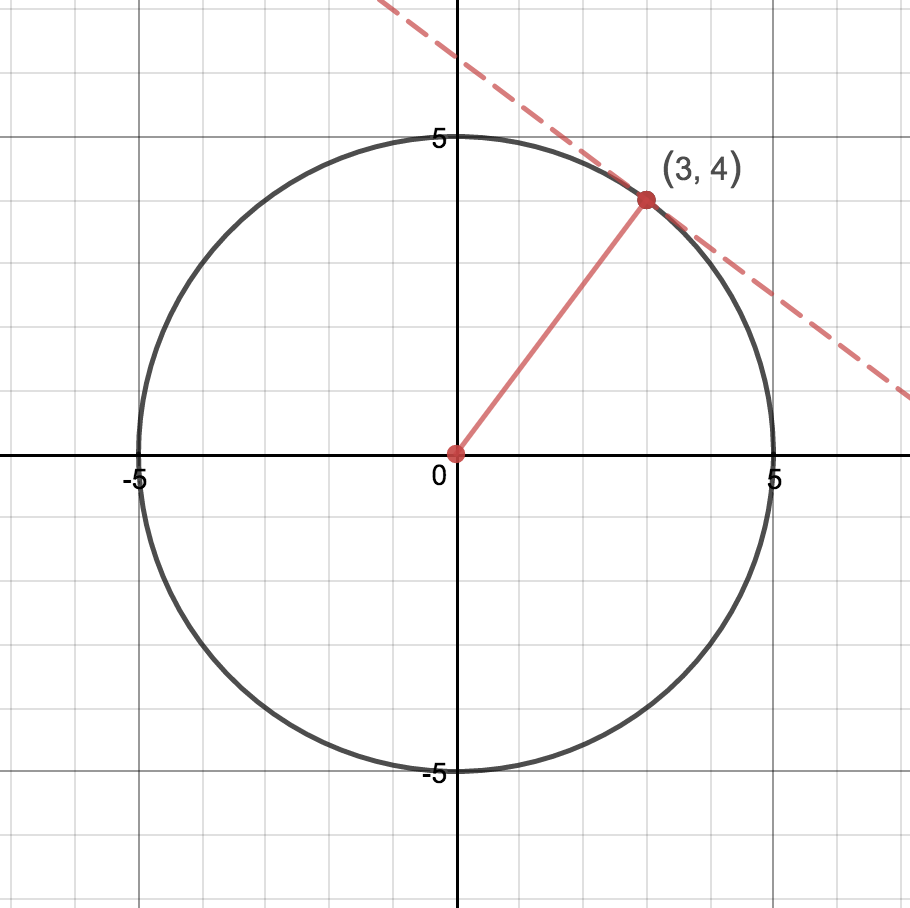### Home > PC > Chapter 9 > Lesson 9.2.1 > Problem9-73

9-73.

Find the equation of the line tangent to the circle x2 + y2 = 25 at the point (3, 4). Homework Help ✎The radius is perpendicular to the tangent line. Find the slope of the radius to help find the slope of the tangent line.

$m_{\text{radius}}=\frac{4}{3}\text{ } m_{\text{tangent}}=-\frac{3}{4}$

Use the given point and mtangent to find the equation.